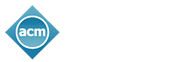#### A PHP Error was encountered

Severity: 8192

Message: Non-static method URL_tube::usage() should not be called statically, assuming \$this from incompatible context

Filename: url_tube/pi.url_tube.php

Line Number: 13

KDD 2020 | AutoShuffleNet: Learning Permutation Matrices via an Exact Lipschitz Continuous Penalty in Deep Convolutional Neural Networks

## AutoShuffleNet: Learning Permutation Matrices via an Exact Lipschitz Continuous Penalty in Deep Convolutional Neural Networks

Jiancheng Lyu: Qualcomm; Shuai Zhang: Qualcomm; Yingyong Qi: Qualcomm; Jack Xin: University of California, Irvine

ShuffleNet is a state-of-the-art light weight convolutional neural network architecture. Its basic operations include group, channel-wise convolution and channel shuffling. However, channel shuffling is manually designed on empirical grounds. Mathematically, shuffling is a multiplication by a permutation matrix. In this paper, we propose to automate channel shuffling by learning permutation matrices in network training. We introduce an exact Lipschitz continuous non-convex penalty so that it can be incorporated in the stochastic gradient descent to approximate permutation at high precision. Exact permutations are obtained by simple rounding at the end of training and are used in inference. The resulting network, referred to as AutoShuffleNet, achieved improved classification accuracies on data from CIFAR-10, CIFAR-100 and ImageNet while preserving the inference costs of ShuffleNet. In addition, we found experimentally that the standard convex relaxation of permutation matrices into stochastic matrices leads to poor performance. We prove theoretically the exactness (error bounds) in recovering permutation matrices when our penalty function is zero (very small). We present examples of permutation optimization through graph matching and two-layer neural network models where the loss functions are calculated in closed analytical form. In the examples, convex relaxation failed to capture permutations whereas our penalty succeeded.

## How can we assist you?

We'll be updating the website as information becomes available. If you have a question that requires immediate attention, please feel free to contact us. Thank you!

Please enter the word you see in the image below: